Algebra

Equations

Inequalities

Graphs

Numbers

Calculus

Matrices

Tutorials

Enter the rational function you want to split into partial fractions, set the options and click the Partial Fractions button.

Expression
Options
 In respect to
Output Style
 Graphic image
 Text

# Using partial fractions to solve integrals

The following are some examples that will demonstrate how partial fraction decomposition is used to solve different types of integrals.

Example 2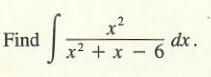Solution Note that the numerator and denominator have the same degree, 2. Dividing x^2 by x2 + x - 6, we obtain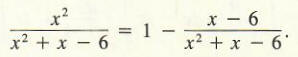For the fraction (x - 6)/(x^2 + x - 6), we now find a partial fraction decomposition as follows.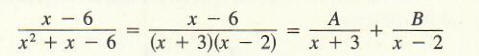Multiplying by (x + 3)(x - 2) gives

x - 6 = A(x - 2) + B(x + 3).

To find A and B, we choose x = 2 and x = - 3 and obtain the following results.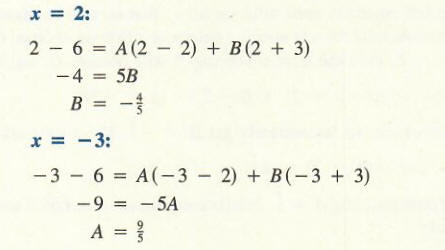Hence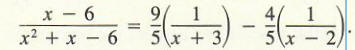To check this, simply add the fractions on the right. The result should be the fraction on the left. Finally, integrate: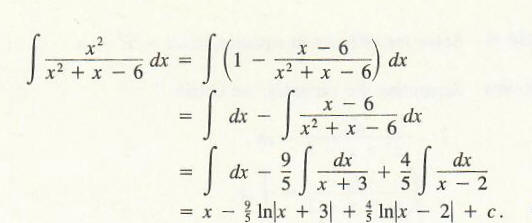The techniques that have been developed extend easily to the case that the denominator is a product of three distinct linear factors. The next example illustrates this

Example 3

Evaluate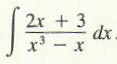Write the integrand as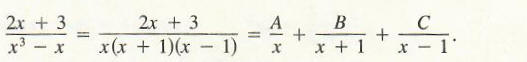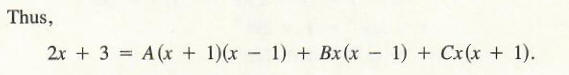For the choices of x below, we obtain the following results: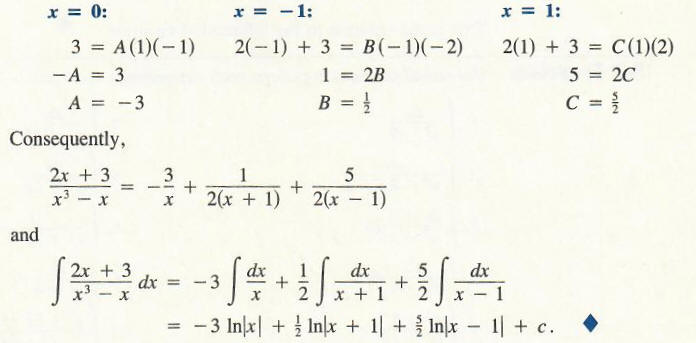In the next section we apply this integration technique to solving the so-called logistic equation. First, let's take a look at some more complex cases of partial fraction decomposition.

## Complex cases of partial fraction decomposition

It is possible for a factor in the denominator to be of higher order. For example, here we have a cube of a binomial present.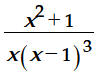Because we can not be sure how the original denominator was created, we have to take into account all possible cases. This means that the following original denominators were possible : (x-1), (x-1)2, (x-1)3   .Consequently, all corresponding fractions need to be included: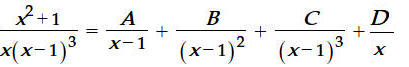After getting rid of denominators the equation looks like this: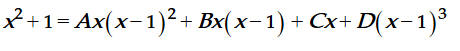The standard way to solve this is making a system of 4 linear equations in A,B,C and D. However this approach gets messy quickly. There is another way to do this - substitute some values for x that will make the equation significantly simpler. The good candidates for x values are 1 and 2. Because most of the equation disappears, this immediately gives us C =2 and D = -1.

We are still not done - A and B need to be solved for. However now we are down to two equations, because we already know C and D. Choosing some other 'simple' values for x, such as 2 and -1 will give us two equations in A and B that are trivial:

A + B = 1 and

2A - B =2

From here, we can easily decompose into the following fractions: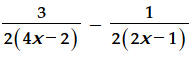Note there are less fractions in the final answer than expected, because one of the constants turned out to be a 0.

It is also possible that denominator is a polynmial in x that can not be factored. In that case you have to account for all possible numerators by creating fractions with all degrees of x less than the denominator. For example, if the denominator is a cubic, you would have to have 3 fractions with numerators 1, x and  xto account for the 3rd degree in the denominator..